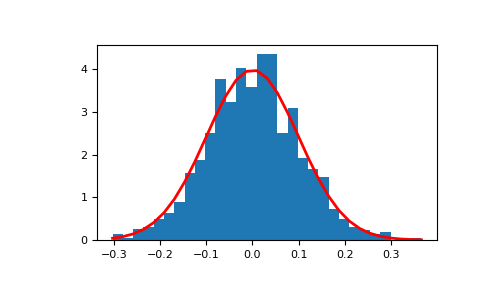numpy.random.Generator.normal¶

method

Generator.normal(loc=0.0, scale=1.0, size=None)

Draw random samples from a normal (Gaussian) distribution.

The probability density function of the normal distribution, first derived by De Moivre and 200 years later by both Gauss and Laplace independently , is often called the bell curve because of its characteristic shape (see the example below).

The normal distributions occurs often in nature. For example, it describes the commonly occurring distribution of samples influenced by a large number of tiny, random disturbances, each with its own unique distribution .

Parameters: loc : float or array_like of floats Mean (“centre”) of the distribution. scale : float or array_like of floats Standard deviation (spread or “width”) of the distribution. Must be non-negative. size : int or tuple of ints, optional Output shape. If the given shape is, e.g., (m, n, k), then m * n * k samples are drawn. If size is None (default), a single value is returned if loc and scale are both scalars. Otherwise, np.broadcast(loc, scale).size samples are drawn. out : ndarray or scalar Drawn samples from the parameterized normal distribution.

scipy.stats.norm
probability density function, distribution or cumulative density function, etc.

Notes

The probability density for the Gaussian distribution iswhereis the mean andthe standard deviation. The square of the standard deviation,, is called the variance.

The function has its peak at the mean, and its “spread” increases with the standard deviation (the function reaches 0.607 times its maximum atand). This implies that normal is more likely to return samples lying close to the mean, rather than those far away.

References

  Wikipedia, “Normal distribution”, https://en.wikipedia.org/wiki/Normal_distribution
  (1, 2, 3) P. R. Peebles Jr., “Central Limit Theorem” in “Probability, Random Variables and Random Signal Principles”, 4th ed., 2001, pp. 51, 51, 125.

Examples

Draw samples from the distribution:

>>> mu, sigma = 0, 0.1 # mean and standard deviation
>>> s = np.random.default_rng().normal(mu, sigma, 1000)

Verify the mean and the variance:

>>> abs(mu - np.mean(s))
0.0  # may vary
>>> abs(sigma - np.std(s, ddof=1))
0.1  # may vary

Display the histogram of the samples, along with the probability density function:

>>> import matplotlib.pyplot as plt
>>> count, bins, ignored = plt.hist(s, 30, density=True)
>>> plt.plot(bins, 1/(sigma * np.sqrt(2 * np.pi)) *
...                np.exp( - (bins - mu)**2 / (2 * sigma**2) ),
...          linewidth=2, color='r')
>>> plt.show()Two-by-four array of samples from N(3, 6.25):

>>> np.random.default_rng().normal(3, 2.5, size=(2, 4))
array([[-4.49401501,  4.00950034, -1.81814867,  7.29718677],   # random
[ 0.39924804,  4.68456316,  4.99394529,  4.84057254]])  # random

Previous topic

numpy.random.Generator.noncentral_f

Next topic

numpy.random.Generator.pareto